Скачать презентацию Uninformed Search Strategies CPSC 322 Search 2

6b5e9daabf74d44a727a12f8e4a0d1ca.ppt

• Количество слайдов: 35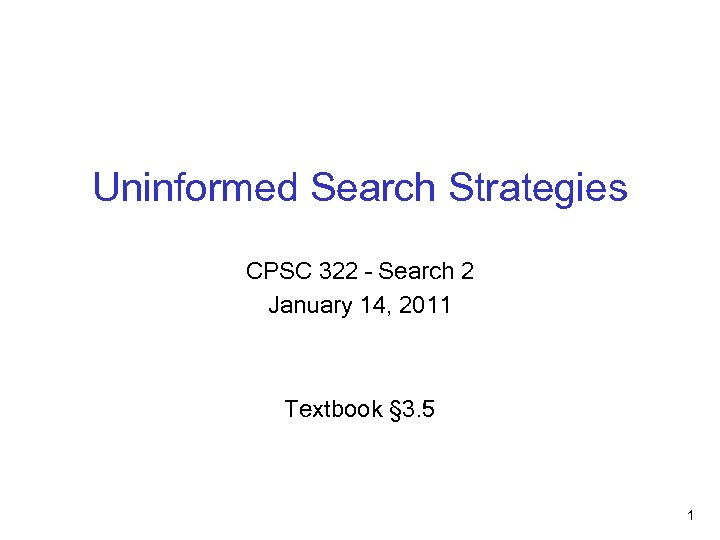Uninformed Search Strategies CPSC 322 – Search 2 January 14, 2011 Textbook § 3. 5 1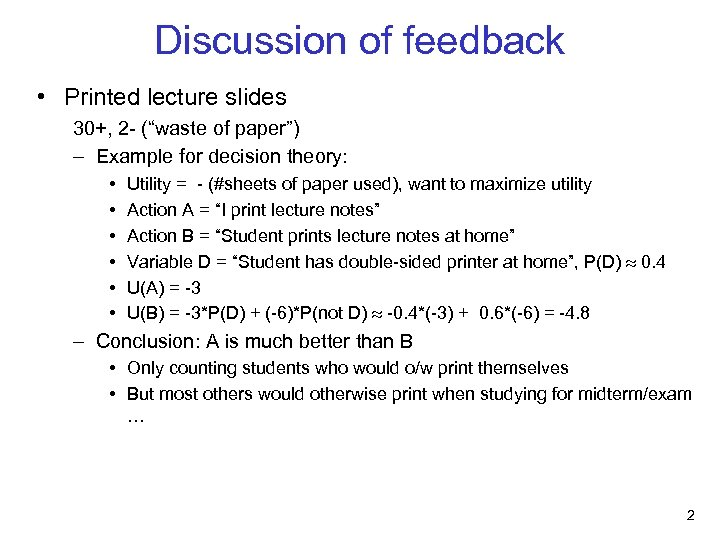Discussion of feedback • Printed lecture slides 30+, 2 - (“waste of paper”) – Example for decision theory: • • • Utility = - (#sheets of paper used), want to maximize utility Action A = “I print lecture notes” Action B = “Student prints lecture notes at home” Variable D = “Student has double-sided printer at home”, P(D) 0. 4 U(A) = -3 U(B) = -3*P(D) + (-6)*P(not D) -0. 4*(-3) + 0. 6*(-6) = -4. 8 – Conclusion: A is much better than B • Only counting students who would o/w print themselves • But most others would otherwise print when studying for midterm/exam … 2Discussion of feedback • Examples: unanimous good 25+, 10 - “more examples”, 3 - “more real-world examples” • Videos: unanimous good Please send me any cool videos you find during the course • Coloured cards: unanimous helpful 23+, 3 - “even more, please” 2 - “most of us have clickers”, 3+ “thanks for NOT using clickers” 3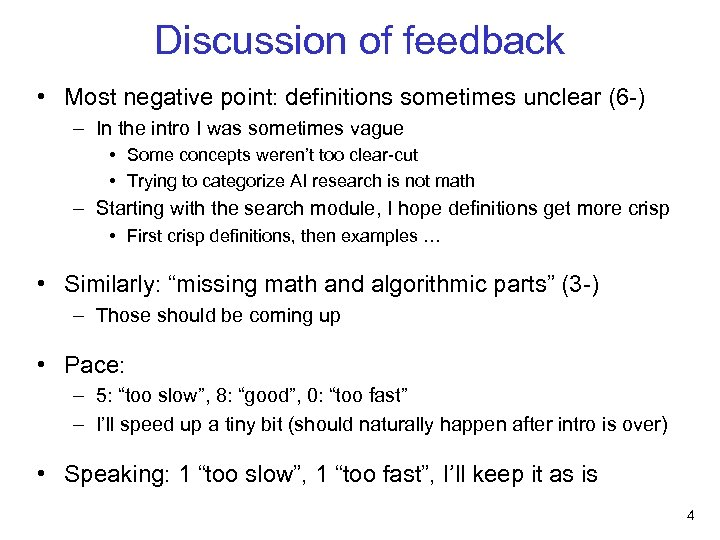Discussion of feedback • Most negative point: definitions sometimes unclear (6 -) – In the intro I was sometimes vague • Some concepts weren’t too clear-cut • Trying to categorize AI research is not math – Starting with the search module, I hope definitions get more crisp • First crisp definitions, then examples … • Similarly: “missing math and algorithmic parts” (3 -) – Those should be coming up • Pace: – 5: “too slow”, 8: “good”, 0: “too fast” – I’ll speed up a tiny bit (should naturally happen after intro is over) • Speaking: 1 “too slow”, 1 “too fast”, I’ll keep it as is 4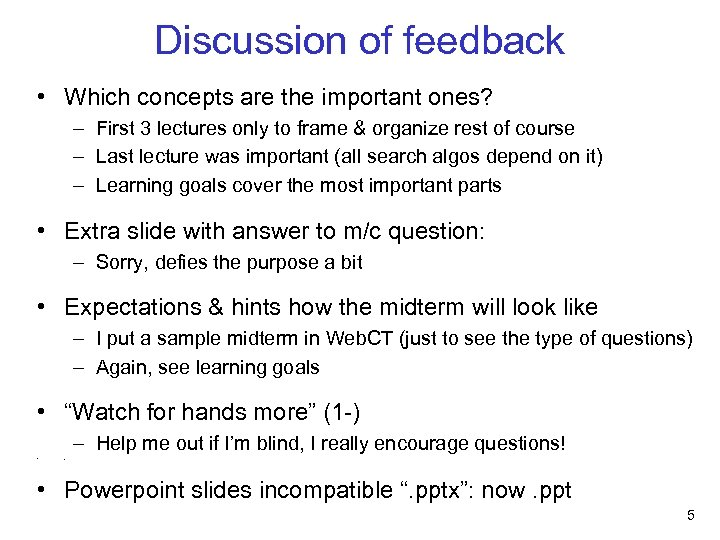Discussion of feedback • Which concepts are the important ones? – First 3 lectures only to frame & organize rest of course – Last lecture was important (all search algos depend on it) – Learning goals cover the most important parts • Extra slide with answer to m/c question: – Sorry, defies the purpose a bit • Expectations & hints how the midterm will look like – I put a sample midterm in Web. CT (just to see the type of questions) – Again, see learning goals • “Watch for hands more” (1 -) – Help me out if I’m blind, I really encourage questions! • < • Powerpoint slides incompatible “. pptx”: now. ppt 5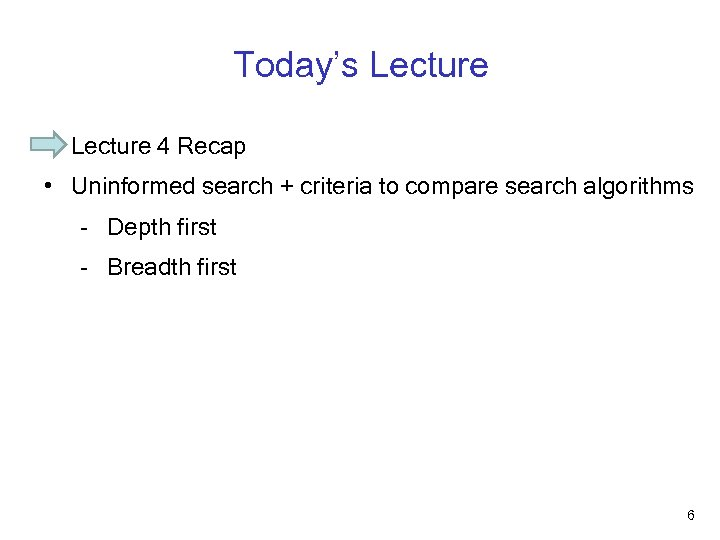Today’s Lecture • Lecture 4 Recap • Uninformed search + criteria to compare search algorithms - Depth first - Breadth first 6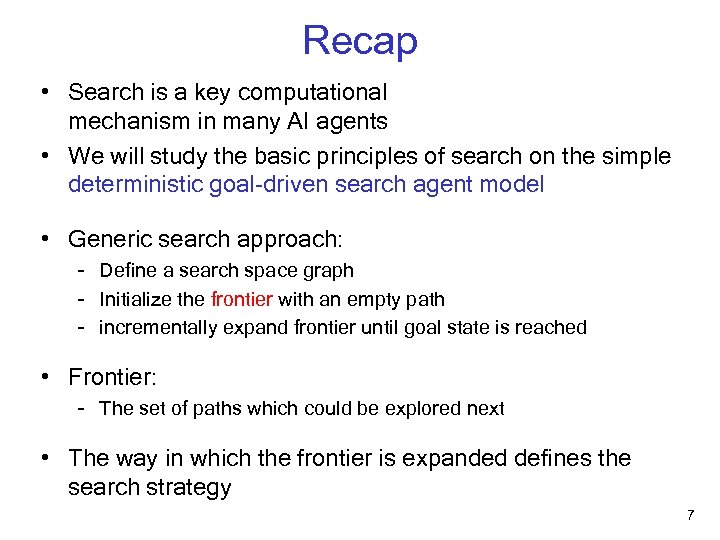Recap • Search is a key computational mechanism in many AI agents • We will study the basic principles of search on the simple deterministic goal-driven search agent model • Generic search approach: - Define a search space graph - Initialize the frontier with an empty path - incrementally expand frontier until goal state is reached • Frontier: - The set of paths which could be explored next • The way in which the frontier is expanded defines the search strategy 7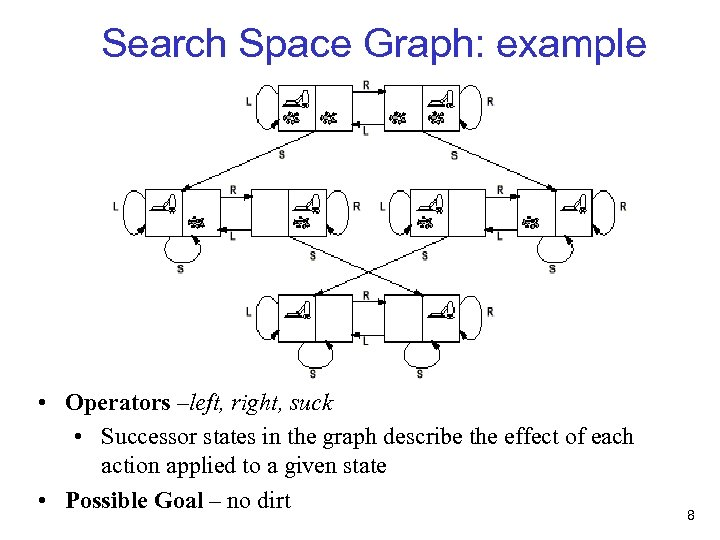Search Space Graph: example • Operators –left, right, suck • Successor states in the graph describe the effect of each action applied to a given state • Possible Goal – no dirt 8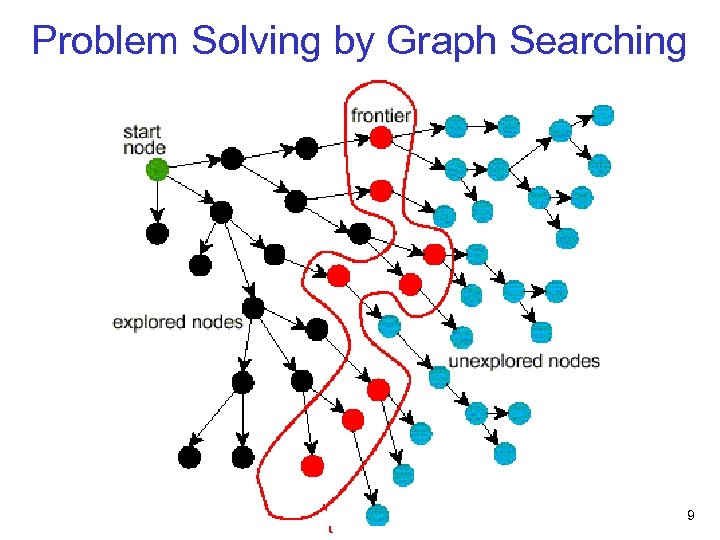Problem Solving by Graph Searching 9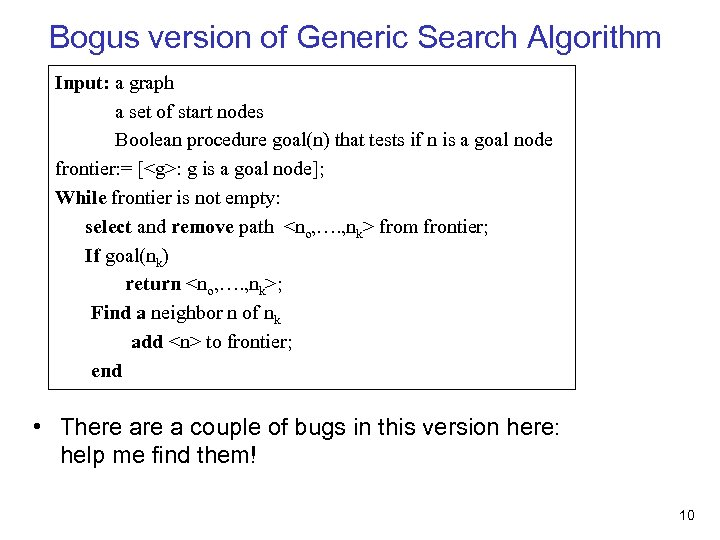Bogus version of Generic Search Algorithm Input: a graph a set of start nodes Boolean procedure goal(n) that tests if n is a goal node frontier: = [: g is a goal node]; While frontier is not empty: select and remove path from frontier; If goal(nk) return ; Find a neighbor n of nk add to frontier; end • There a couple of bugs in this version here: help me find them! 10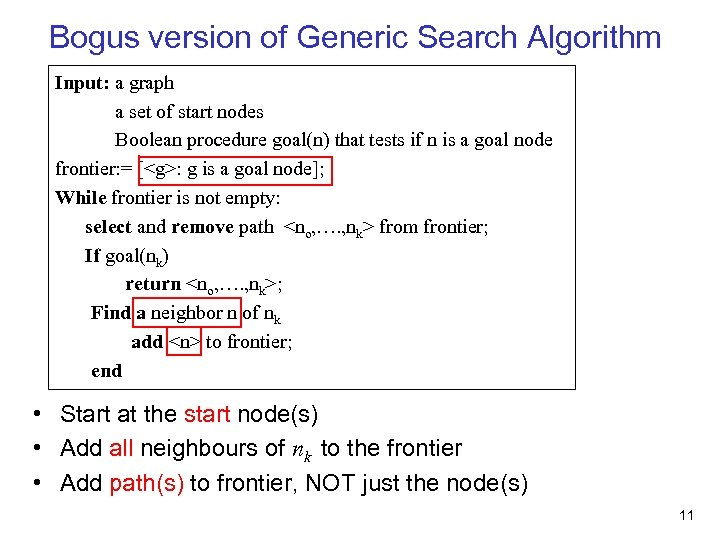Bogus version of Generic Search Algorithm Input: a graph a set of start nodes Boolean procedure goal(n) that tests if n is a goal node frontier: = [: g is a goal node]; While frontier is not empty: select and remove path from frontier; If goal(nk) return ; Find a neighbor n of nk add to frontier; end • Start at the start node(s) • Add all neighbours of nk to the frontier • Add path(s) to frontier, NOT just the node(s) 11Today’s Lecture • Lecture 4 Recap • Uninformed search + criteria to compare search algorithms - Depth first - Breadth first 12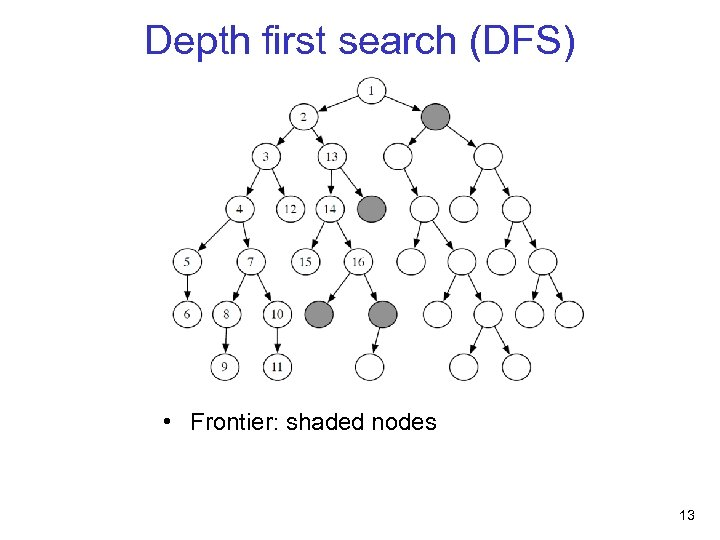Depth first search (DFS) • Frontier: shaded nodes 13Depth first search (DFS) • Frontier: shaded nodes • Which node will be expanded next? (expand = “remove node from frontier & put its successors on”) 14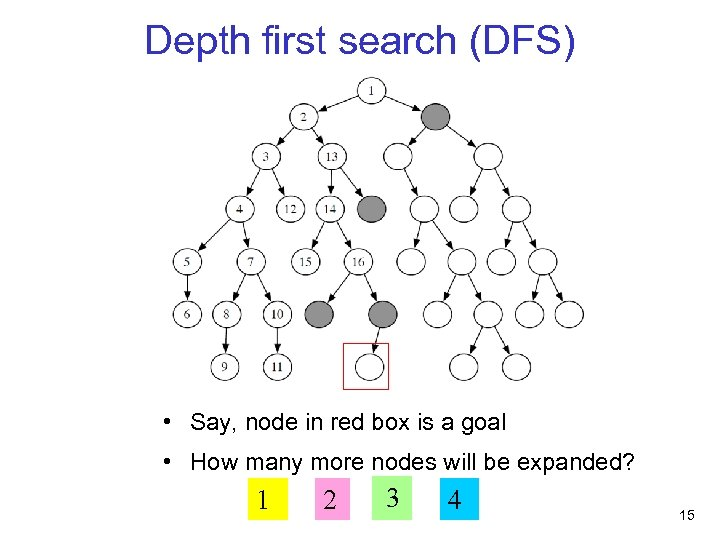Depth first search (DFS) • Say, node in red box is a goal • How many more nodes will be expanded? 1 2 3 4 15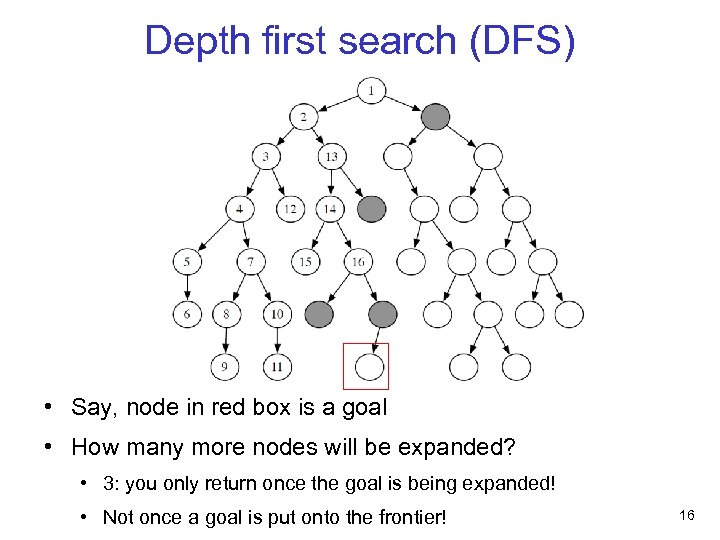Depth first search (DFS) • Say, node in red box is a goal • How many more nodes will be expanded? • 3: you only return once the goal is being expanded! • Not once a goal is put onto the frontier! 16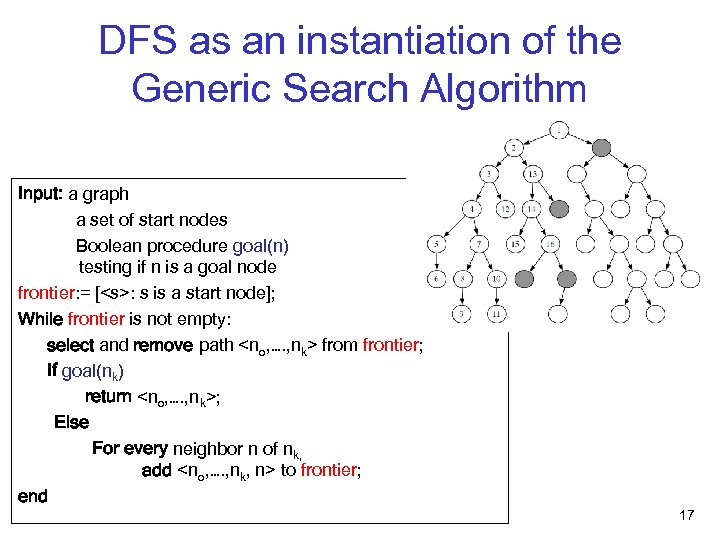DFS as an instantiation of the Generic Search Algorithm Input: a graph a set of start nodes Boolean procedure goal(n) testing if n is a goal node frontier: = [: s is a start node]; While frontier is not empty: select and remove path from frontier; If goal(nk) return ; Else For every neighbor n of nk, add to frontier; end 17DFS as an instantiation of the Generic Search Algorithm Input: a graph a set of start nodes Boolean procedure goal(n) testing if n is a goal node frontier: = [: s is a start node]; While frontier is not empty: select and remove path from frontier; If goal(nk) return ; Else For every neighbor n of nk, add to frontier; end In DFS, the frontier is a last-in-first-out stack 18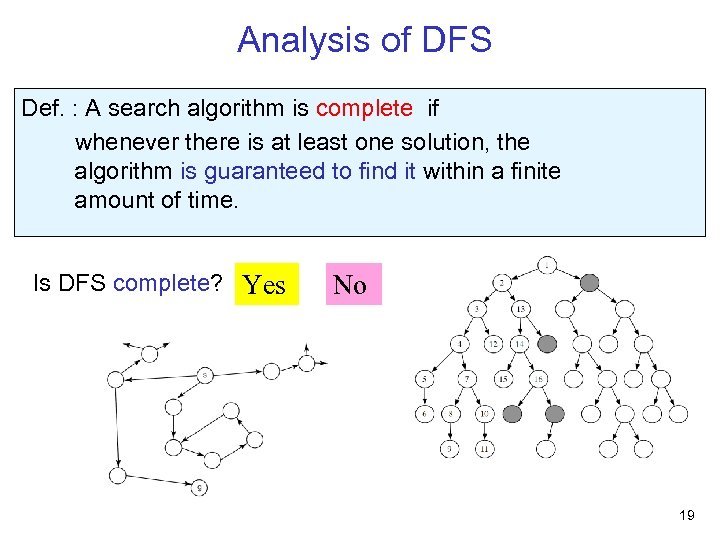Analysis of DFS Def. : A search algorithm is complete if whenever there is at least one solution, the algorithm is guaranteed to find it within a finite amount of time. Is DFS complete? Yes No 19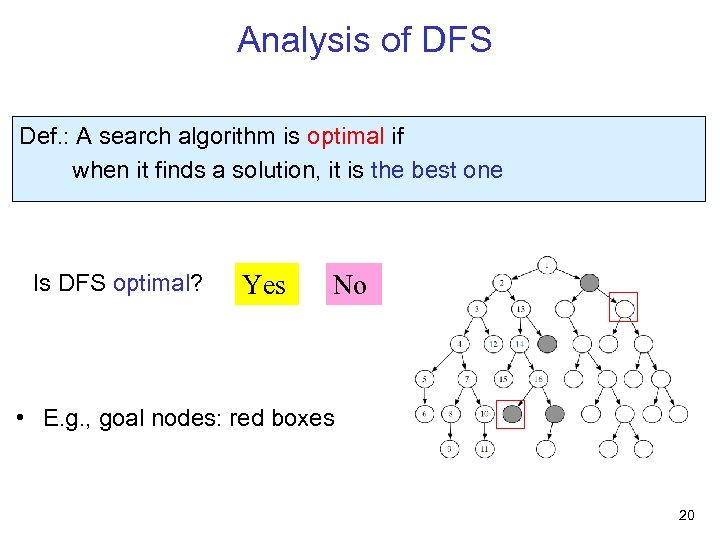Analysis of DFS Def. : A search algorithm is optimal if when it finds a solution, it is the best one Is DFS optimal? Yes No • E. g. , goal nodes: red boxes 20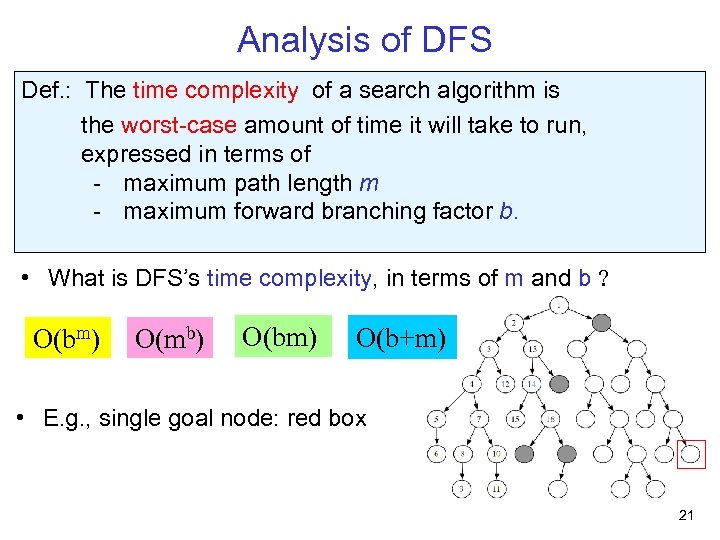Analysis of DFS Def. : The time complexity of a search algorithm is the worst-case amount of time it will take to run, expressed in terms of - maximum path length m - maximum forward branching factor b. • What is DFS’s time complexity, in terms of m and b ? O(bm) O(mb) O(bm) O(b+m) • E. g. , single goal node: red box 21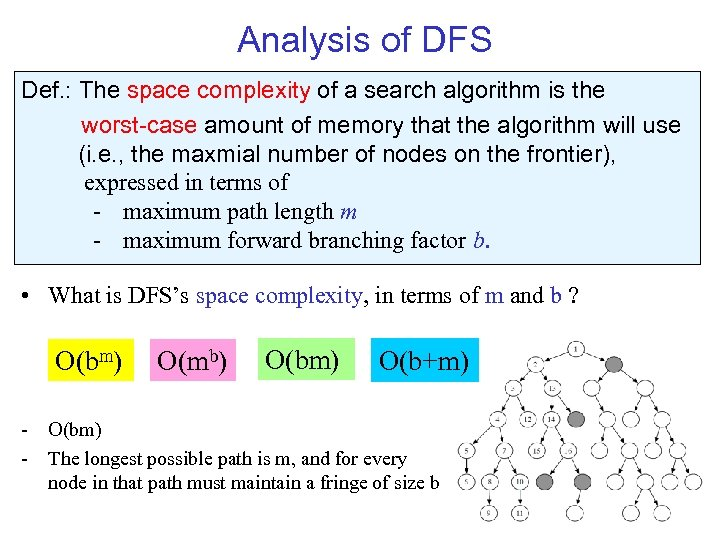Analysis of DFS Def. : The space complexity of a search algorithm is the worst-case amount of memory that the algorithm will use (i. e. , the maxmial number of nodes on the frontier), expressed in terms of - maximum path length m - maximum forward branching factor b. • What is DFS’s space complexity, in terms of m and b ? O(bm) O(mb) O(bm) O(b+m) - O(bm) - The longest possible path is m, and for every node in that path must maintain a fringe of size b 22Today’s Lecture • Lecture 4 Recap • Uninformed search + criteria to compare search algorithms - Depth first - Breadth first 23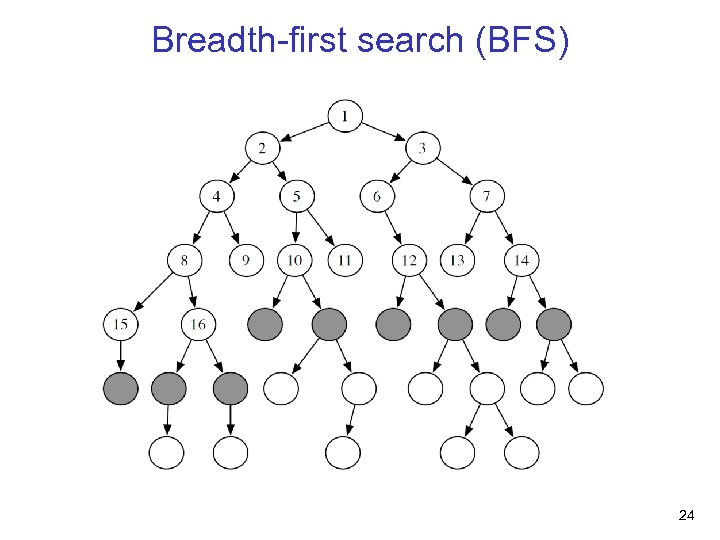Breadth-first search (BFS) 24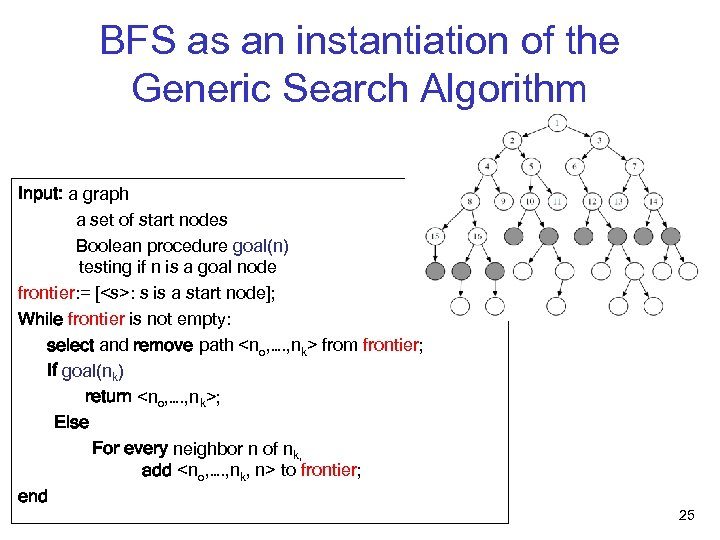BFS as an instantiation of the Generic Search Algorithm Input: a graph a set of start nodes Boolean procedure goal(n) testing if n is a goal node frontier: = [: s is a start node]; While frontier is not empty: select and remove path from frontier; If goal(nk) return ; Else For every neighbor n of nk, add to frontier; end 25BFS as an instantiation of the Generic Search Algorithm Input: a graph a set of start nodes Boolean procedure goal(n) testing if n is a goal node frontier: = [: s is a start node]; While frontier is not empty: select and remove path from frontier; If goal(nk) return ; Else For every neighbor n of nk, add to frontier; end In BFS, the frontier is a first-in-first-out queue 26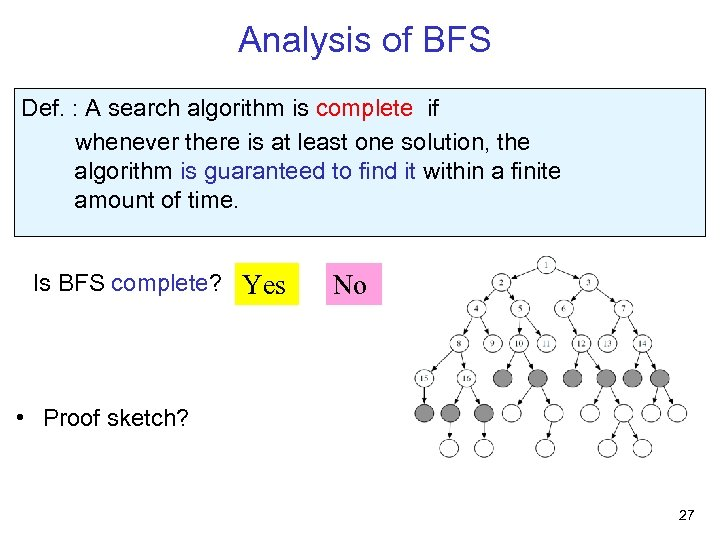Analysis of BFS Def. : A search algorithm is complete if whenever there is at least one solution, the algorithm is guaranteed to find it within a finite amount of time. Is BFS complete? Yes No • Proof sketch? 27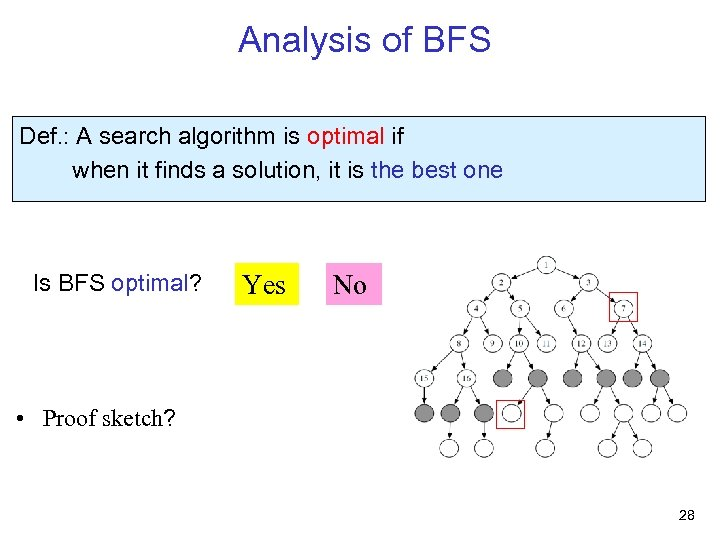Analysis of BFS Def. : A search algorithm is optimal if when it finds a solution, it is the best one Is BFS optimal? Yes No • Proof sketch? 28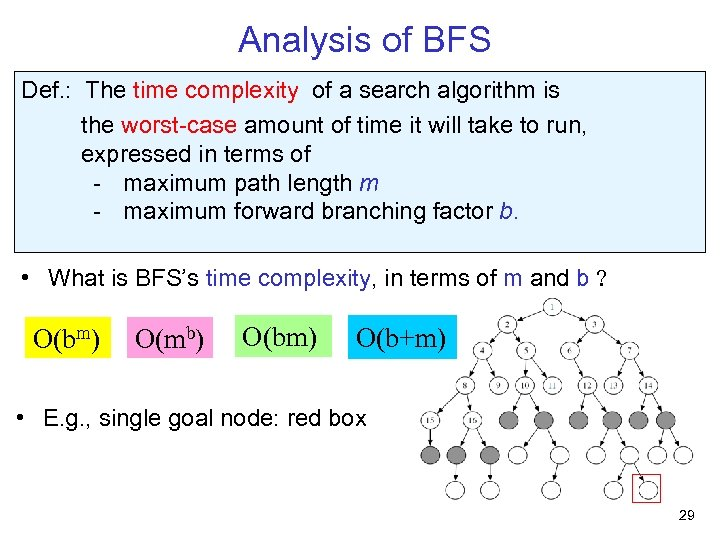Analysis of BFS Def. : The time complexity of a search algorithm is the worst-case amount of time it will take to run, expressed in terms of - maximum path length m - maximum forward branching factor b. • What is BFS’s time complexity, in terms of m and b ? O(bm) O(mb) O(bm) O(b+m) • E. g. , single goal node: red box 29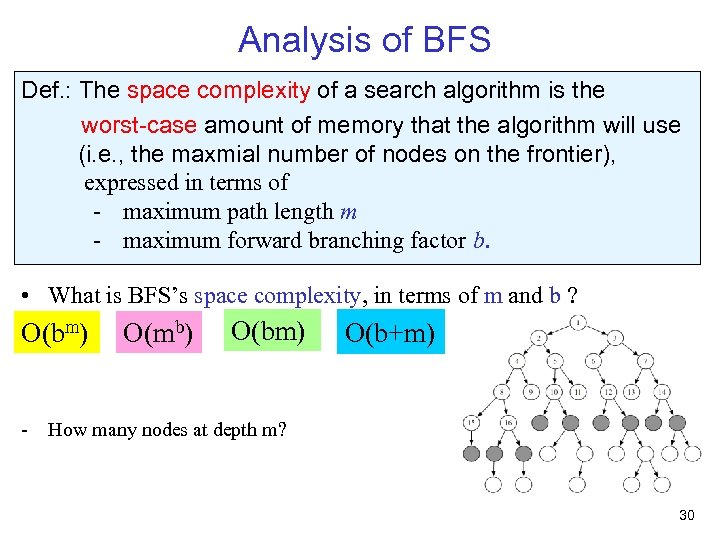Analysis of BFS Def. : The space complexity of a search algorithm is the worst-case amount of memory that the algorithm will use (i. e. , the maxmial number of nodes on the frontier), expressed in terms of - maximum path length m - maximum forward branching factor b. • What is BFS’s space complexity, in terms of m and b ? O(bm) O(mb) O(bm) O(b+m) - How many nodes at depth m? 30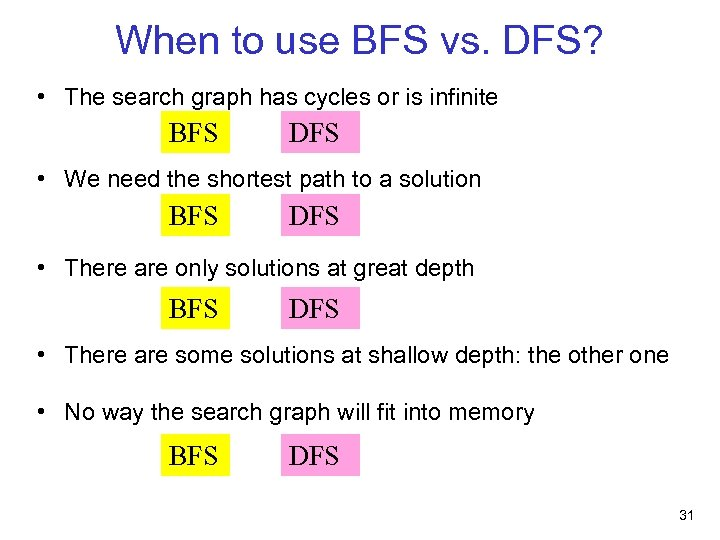When to use BFS vs. DFS? • The search graph has cycles or is infinite BFS DFS • We need the shortest path to a solution BFS DFS • There are only solutions at great depth BFS DFS • There are some solutions at shallow depth: the other one • No way the search graph will fit into memory BFS DFS 31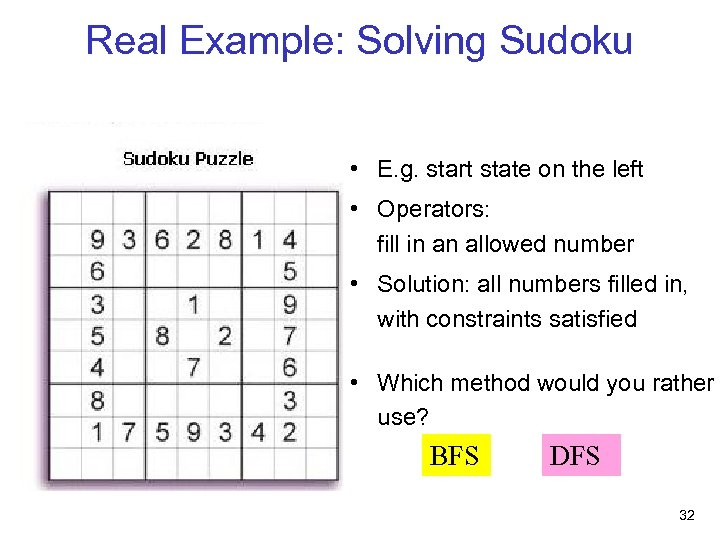Real Example: Solving Sudoku • E. g. start state on the left • Operators: fill in an allowed number • Solution: all numbers filled in, with constraints satisfied • Which method would you rather use? BFS DFS 32Real Example: Eight Puzzle. DFS or BFS? • Which method would you rather use? BFS DFS 33Learning Goals for today’s class • Apply basic properties of search algorithms: - completeness - optimality - time and space complexity of search algorithms • Select the most appropriate search algorithms for specific problems. – Depth-First Search vs. Breadth-First Search 34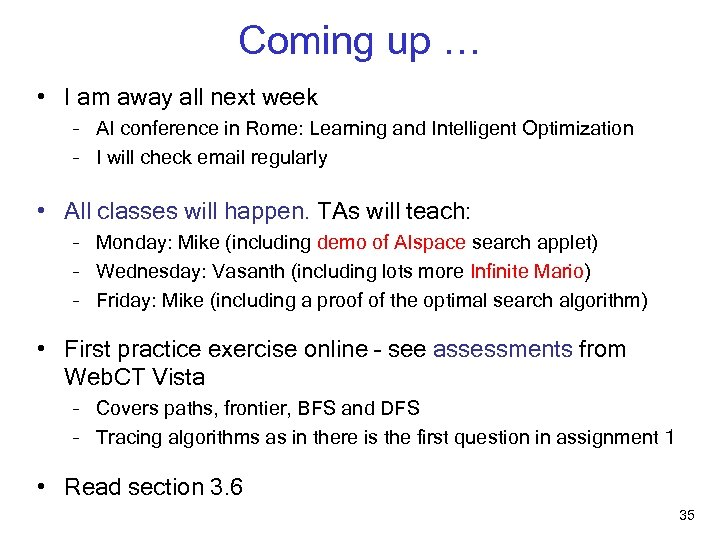Coming up … • I am away all next week – AI conference in Rome: Learning and Intelligent Optimization – I will check email regularly • All classes will happen. TAs will teach: – Monday: Mike (including demo of AIspace search applet) – Wednesday: Vasanth (including lots more Infinite Mario) – Friday: Mike (including a proof of the optimal search algorithm) • First practice exercise online – see assessments from Web. CT Vista – Covers paths, frontier, BFS and DFS – Tracing algorithms as in there is the first question in assignment 1 • Read section 3. 6 35